João Pranke
Subscriber

Of course!
The line voltage is 36.225 kV;
With the external circuit: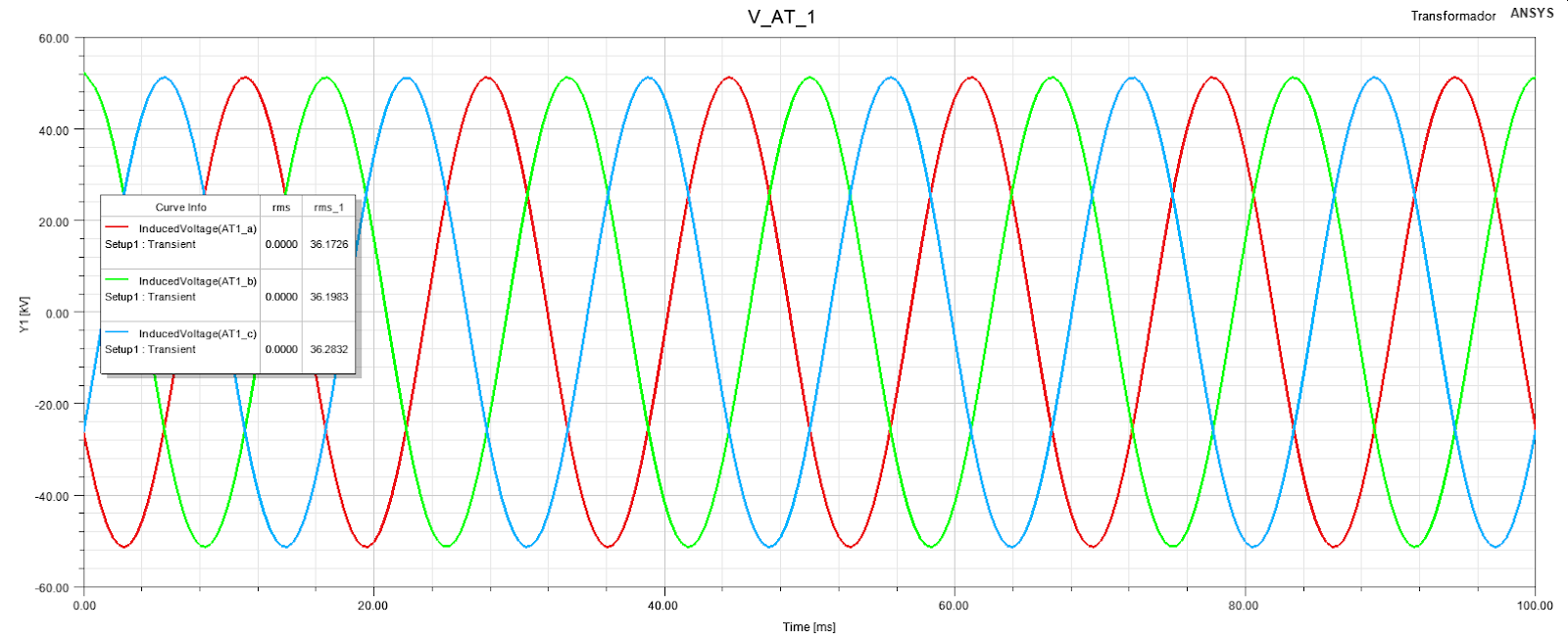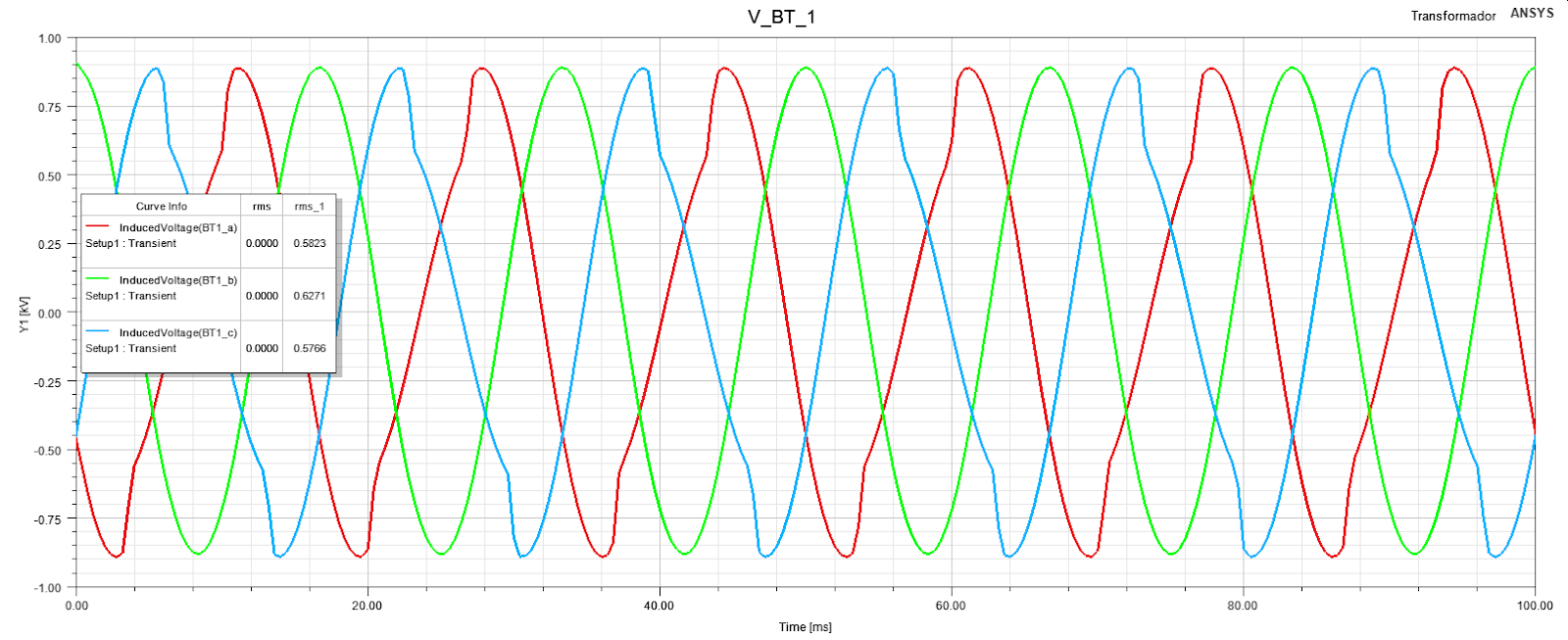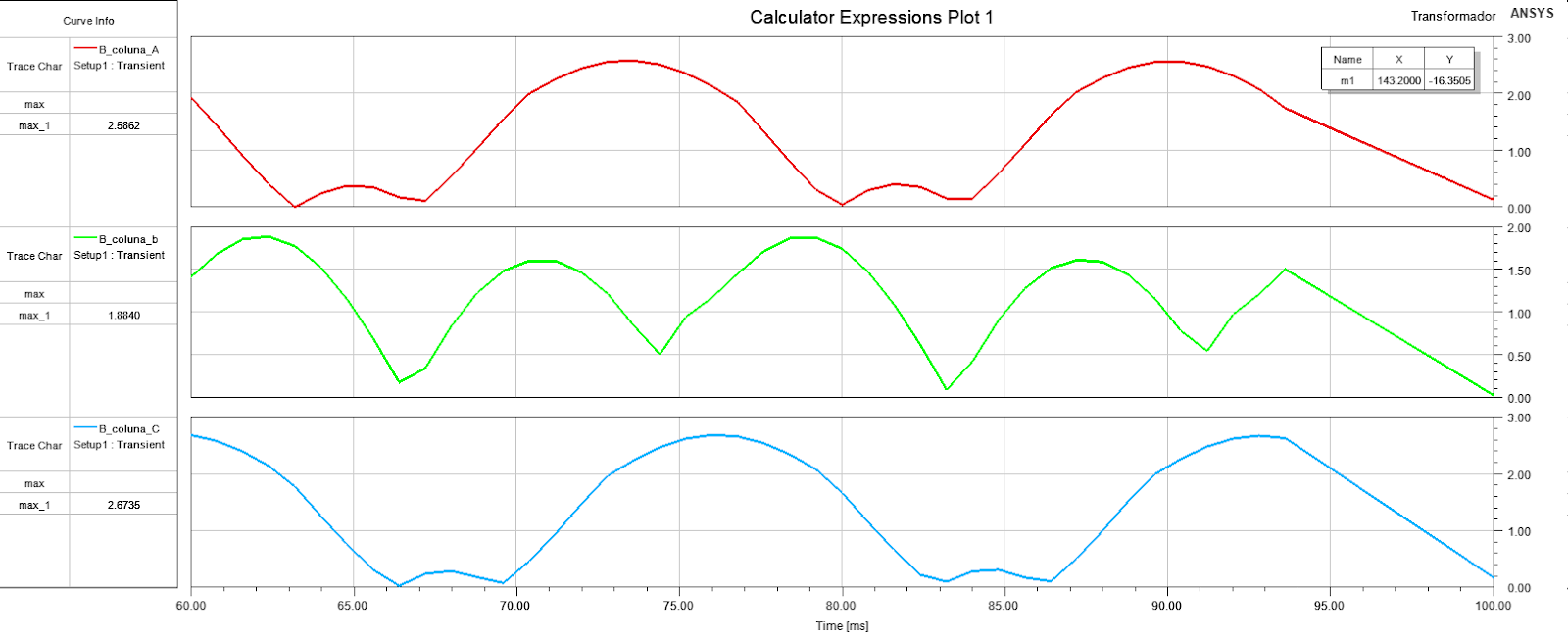(This last graph is the max amplitude of the flux density B on sections of core legs)

Without the external circuit:

The excitation on the widings is the following (AT1 and AT2 are in parallel):

AT1_a=36225*sqrt(2)*cos(2*pi*60*time)

AT1_b=36225*sqrt(2)*cos(2*pi*60*time+120deg)

AT1_c=36225*sqrt(2)*cos(2*pi*60*time+240deg)

AT2_a=36225*sqrt(2)*cos(2*pi*60*time)

AT2_b=36225*sqrt(2)*cos(2*pi*60*time+120deg)

AT2_c=36225*sqrt(2)*cos(2*pi*60*time+240deg)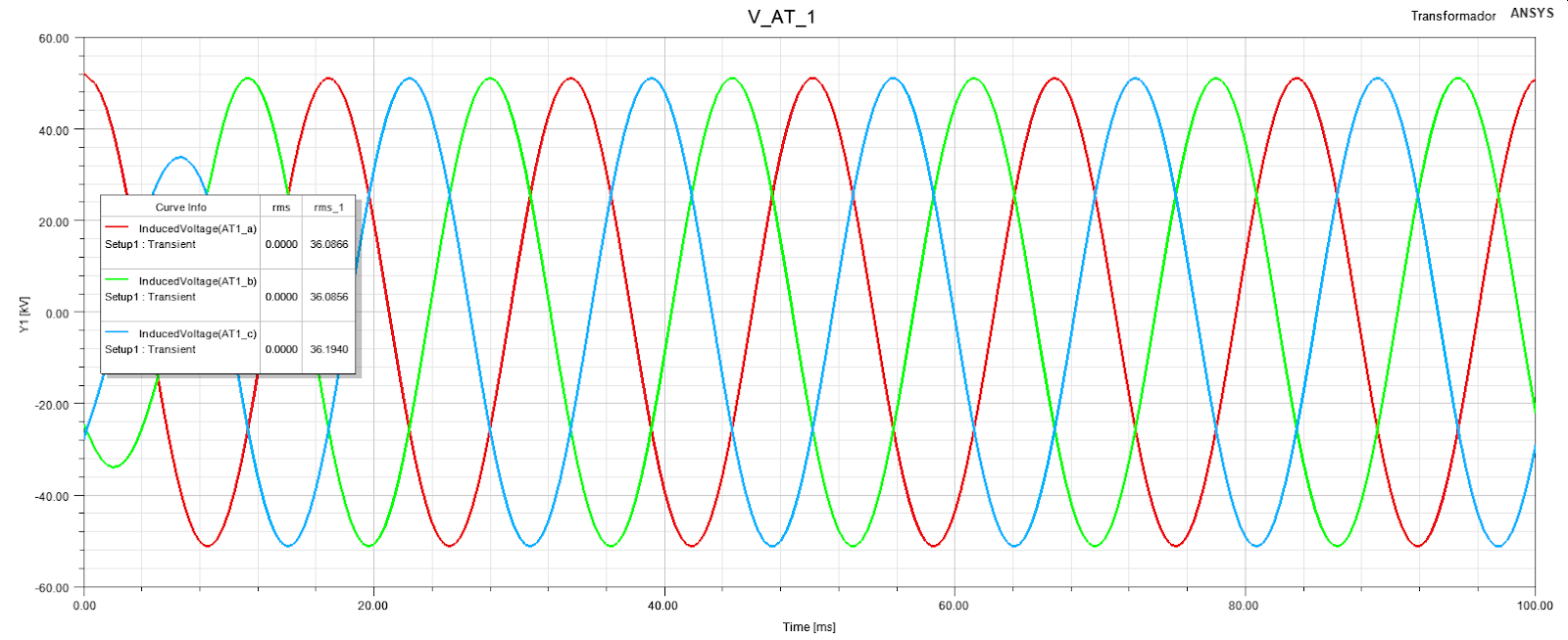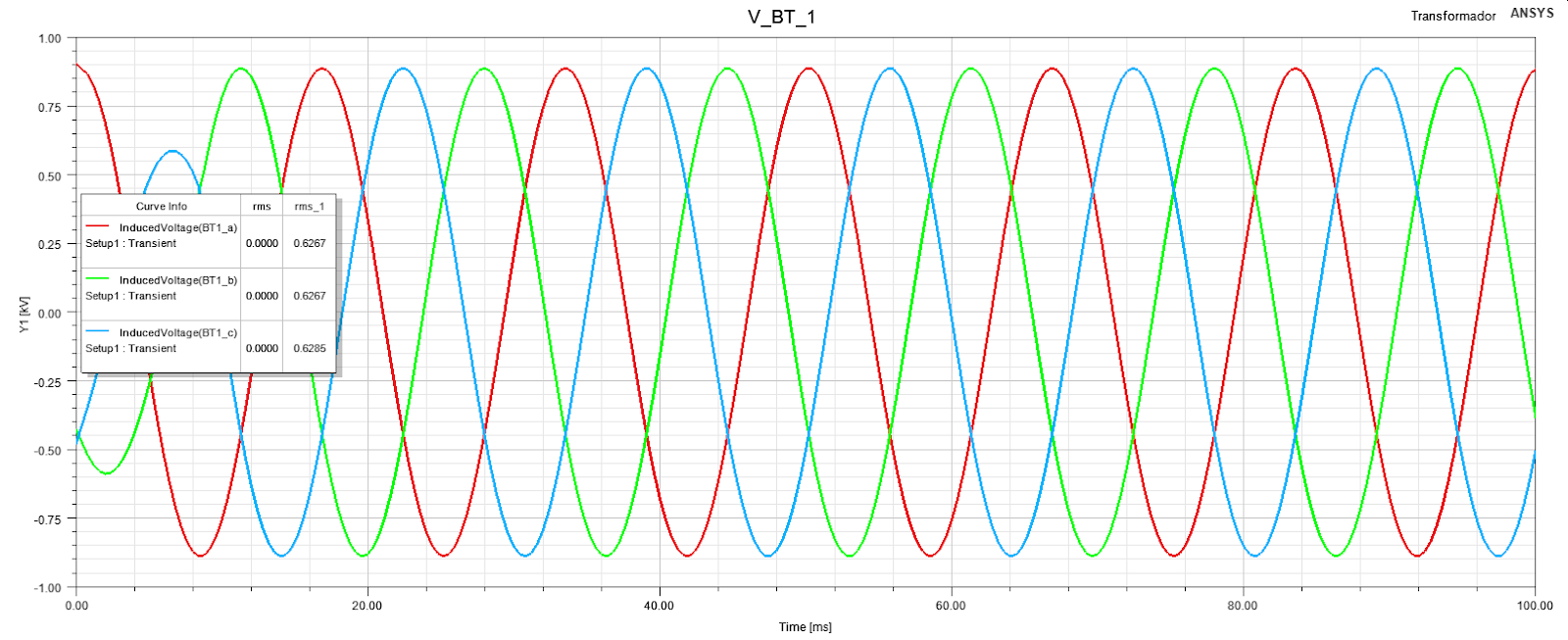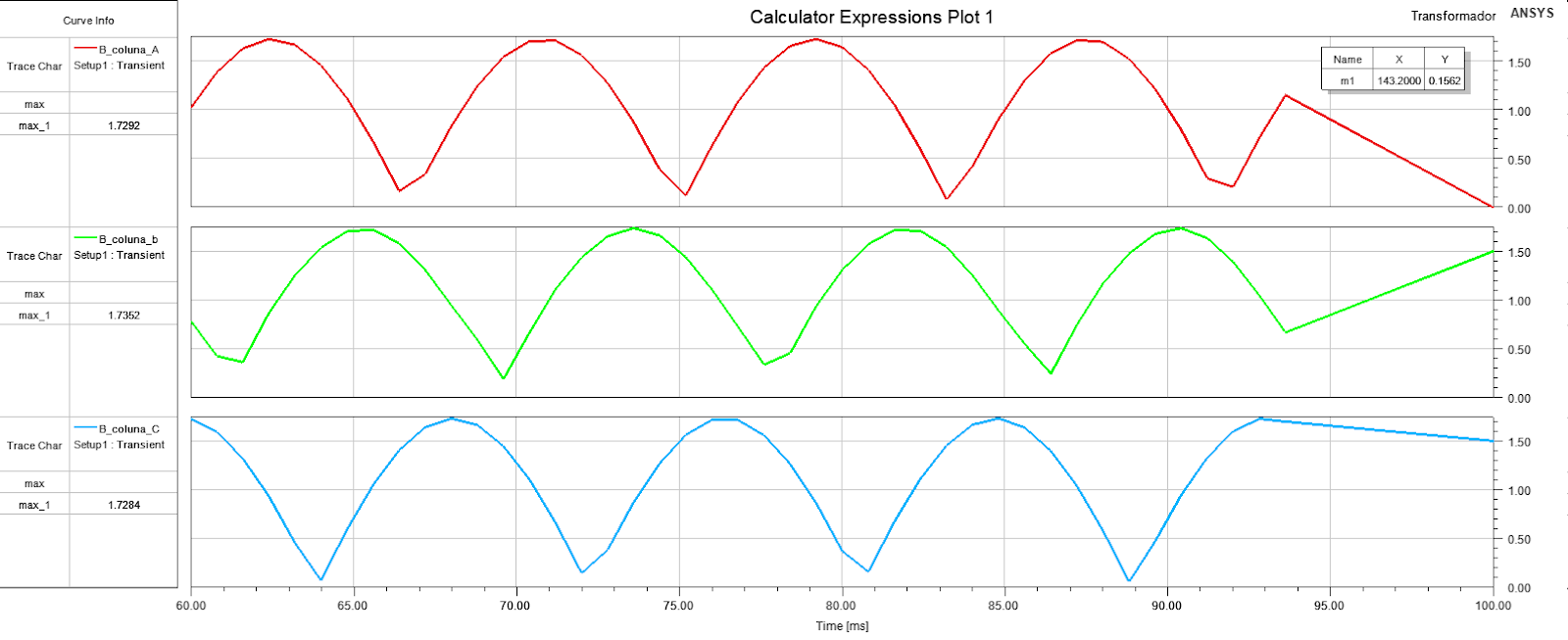Here the flux density is almost exactly of the project (1,73 T)

As i can see, the excitation is exactly the same with or without the external circuit, but the simulation does't work with the circuit.